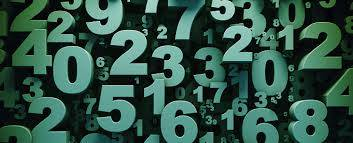How to use compatible numbers to find two estimates?

Using compatible numbers in a sum is a good way of getting a usable estimate when the sum is too complex to perform in your head, or you don't need to be completely accurate with the answer.

This usually means making the largest number in the sum easily divisible by the smaller number.For example, if you are given the sum 11972/6 = ?, you can make the calculation easier by taking 11972 and rounding it up to the nearest hundred. This would give us 12000.

12 is easy to divide by 6 in our heads, as we know 12/6 = 2. So, 12000/6 is just a case of 12/6 and adding the 000s (hundreds/tens/units) once we've done. Therefore:

12000/6 = 2000.

This doesn't tell us the exact answer to 11972/6, but 2000 is a workable estimate. This estimate is known as a quotient.

As your question suggests, we can use another estimate to get a closer figure, while still keeping things simple. We could turn 11972 into 9072. This looks tricky, but if we stick to 6 as a divisor, it isn't so bad. Break the number 9072 into 2 pieces:

90/6 = 15

72/6 = 12

Therefore 9072/6 = 1512

With this, we know the answer to 11972/6 is somewhere between the quotients of 2000 and 1512, and is much closer to 2000 than 1512. You can experiment yourself to find a closer range of quotients.

This method can also be used with addition, subtraction and multiplication. You can find further examples at virtualnerd.com/tutorial or basic-mathematics.com/what-are-compatible-numbers.

YouTube is a good place to look for maths tutorials, and here's one YouTube user to show you how to use compatible numbers:

thanked the writer.